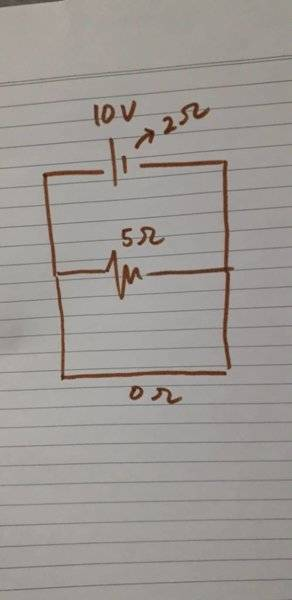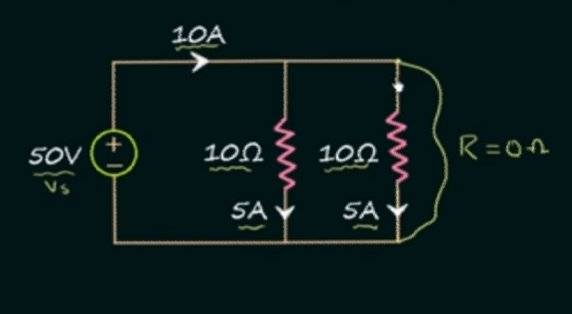# What is the current flowing in this zero-Ohm path?

Kevin J
The 2 Ohms on the voltage source is its internal resistance, what is the current flowing through the (0 Ohms) Path?#### Attachments

Mentor
Is this for schoolwork? What do you think the answer would be?

•Kevin J
Mentor
Hint, for a voltage source the 2 Ohm internal resistance is in series with the 10 V.

•Kevin J
Kevin J
Is this for schoolwork? What do you think the answer would be?
Don't worry, this is not a school hw, shouldn't the current be 5A? Since the current only flows through the 0 Ohms path?

Kevin J
Hint, for a voltage source the 2 Ohm internal resistance is in series with the 10 V.
Ok, let me think, if the parallel resistance is (5×0)/5+0, this means the parallel resistance is equal to 0 Ohms, if the 2 Ohm resistance is series doesn't that mean 0+2 Ohms? Correct me if I'm wrong

Mentor
shouldn't the current be 5A?
Yes, exactly!

•Kevin J
Kevin J
Yes, exactly!
Thanks! People here are so nice, in physicsstackexchange, they are sometimes very rude, every question I asked is considered as a duplicate and they even banned me from their forum :(

•Dale
Kevin J
Then what about this, I saw this video from youtube, is this even correct? Shouldn't the resistance being considered in the calculation, is the internal resistance of the battery? View attachment 233635

Kevin J
The person on the web says the 10A flows through the short circuit(0 resistance path), but how do you even get this conculsion? If we take a look at the problem, the total parallel resistance would be (10×10×0)/10+10+0=0 Ohms, using V=I×R, doesn't it mean current is infinite?
*
To make it clearer if you look at the diagram drawn by me(brown pen), the current flowing through the short circuit path would be 5A, because the source has internal resistance (10V/2Ohms), it is very clear that I don't use the 5 Ohms resistor to calculate the current, so why do the person on the web used those resistors?#### Attachments

Homework Helper
Gold Member
In my opinion you are right that the current will be infinite.

BUT perhaps the person on the web says something else which you didn't notice, perhaps he is saying that the voltage source has internal resistance 5Ohm?

OR perhaps if originally the circuit is not shorted, then we have 10A current flowing. When we short circuit it, then there will be a small transient state during which the current will rise from 10A to infinite. At the start of the transient state the current flowing through the short circuit will be 10A and soon it will become infinite. Is this what the person at the web says?

Gold Member
2022 Award
The person on the web
Which person? Can you post a link to the video?

Kevin J
Which person? Can you post a link to the video?

Here's the link, was there something I missed?

Homework Helper
Gold Member
Ok, well the video discriminates between two cases:

The ideal case: I agree with the video that current will be infinite, because an ideal source can keep steady voltage even for infinite current through the source.

The practical case: I partially agree with the video. The current in this case will be finite(it will not be 10A as the video says) but very large, it will start from 10A and rise to the large finite value (which will be the maximum current that the non ideal voltage source supports). Then one of the following things can happen because the voltage source is not ideal and can support up to a maximum value of current
1) The voltage source might catch fire
2) The voltage source might drop its voltage and so the current will drop back to a lower value
3) If the voltage source is protected, then just the fuse will intervene and the current will drop to zero
4) In general some other unpredictable thing , like the short circuit path catching fire (because it will become extremely hot due to the large current) e.t.c

•Wrichik Basu
Homework Helper
Gold Member
There are at least two problems with his explanation of the practical case. (I'm ignoring super conductors here)..

1) All real world voltage sources have some internal resistance and he should show that on his circuit.

2) All real world short circuits have some resistance and he should show that on his circuit.

It is the combination of these two resistances that determines/limits how much current will flow.

Edit: Delta mentions other reasons why a practical circuit might behave differently that are also correct.

•OmCheeto and Delta2
Gold Member
2022 Award
Thanks! People here are so nice, in physicsstackexchange, they are sometimes very rude, every question I asked is considered as a duplicate and they even banned me from their forum :(
Same reason why I sought refuge here in PF. I still have an account in physics StackExchange, but I go there once in a long while to chat with old friends who are reluctant to come here.

Kevin J
Ok, well the video discriminates between two cases:

The ideal case: I agree with the video that current will be infinite, because an ideal source can keep steady voltage even for infinite current through the source.

The practical case: I partially agree with the video. The current in this case will be finite(it will not be 10A as the video says) but very large, it will start from 10A and rise to the large finite value (which will be the maximum current that the non ideal voltage source supports). Then one of the following things can happen because the voltage source is not ideal and can support up to a maximum value of current
1) The voltage source might catch fire
2) The voltage source might drop its voltage and so the current will drop back to a lower value
3) If the voltage source is protected, then just the fuse will intervene and the current will drop to zero
4) In general some other unpredictable thing , like the short circuit path catching fire (because it will become extremely hot due to the large current) e.t.c
and how do you count the battery's maximum current, is it it's voltage divided by it's internal resistance?

Homework Helper
Gold Member
and how do you count the battery's maximum current, is it it's voltage divided by it's internal resistance?
Maybe you could take as an upper bound that value (EMF/internal resistance) , because for this value the voltage drop at the battery's terminals will become zero but I think most batteries can support maximum current that it is quite smaller than this value.

Mentor
The person on the web says the 10A flows through the short circuit(0 resistance path), but how do you even get this conculsion? If we take a look at the problem, the total parallel resistance would be (10×10×0)/10+10+0=0 Ohms...
Try that math again...

Kevin J
Try that math again...
What do you mean try the math again?0 divided by 20 is 0

Mentor
What do you mean try the math again?0 divided by 20 is 0
0+10 is 10

Unless you wrote it wrong, you're doing the order of operations wrong.

Homework Helper
Gold Member
Try that math again...
The usual problem when we don't use latex, we forget to put parentheses and this can mess a lot things. He means (10x10x0)/(10+10+0)

Though again for three resistances in parallel the total resistance is ##\frac{R_1R_2R_3}{R_1R_2+R_1R_3+R_2R_3}## in this case it should be ##\frac{10\cdot10\cdot0}{10\cdot10+10\cdot0+10\cdot0}##

•russ_watters